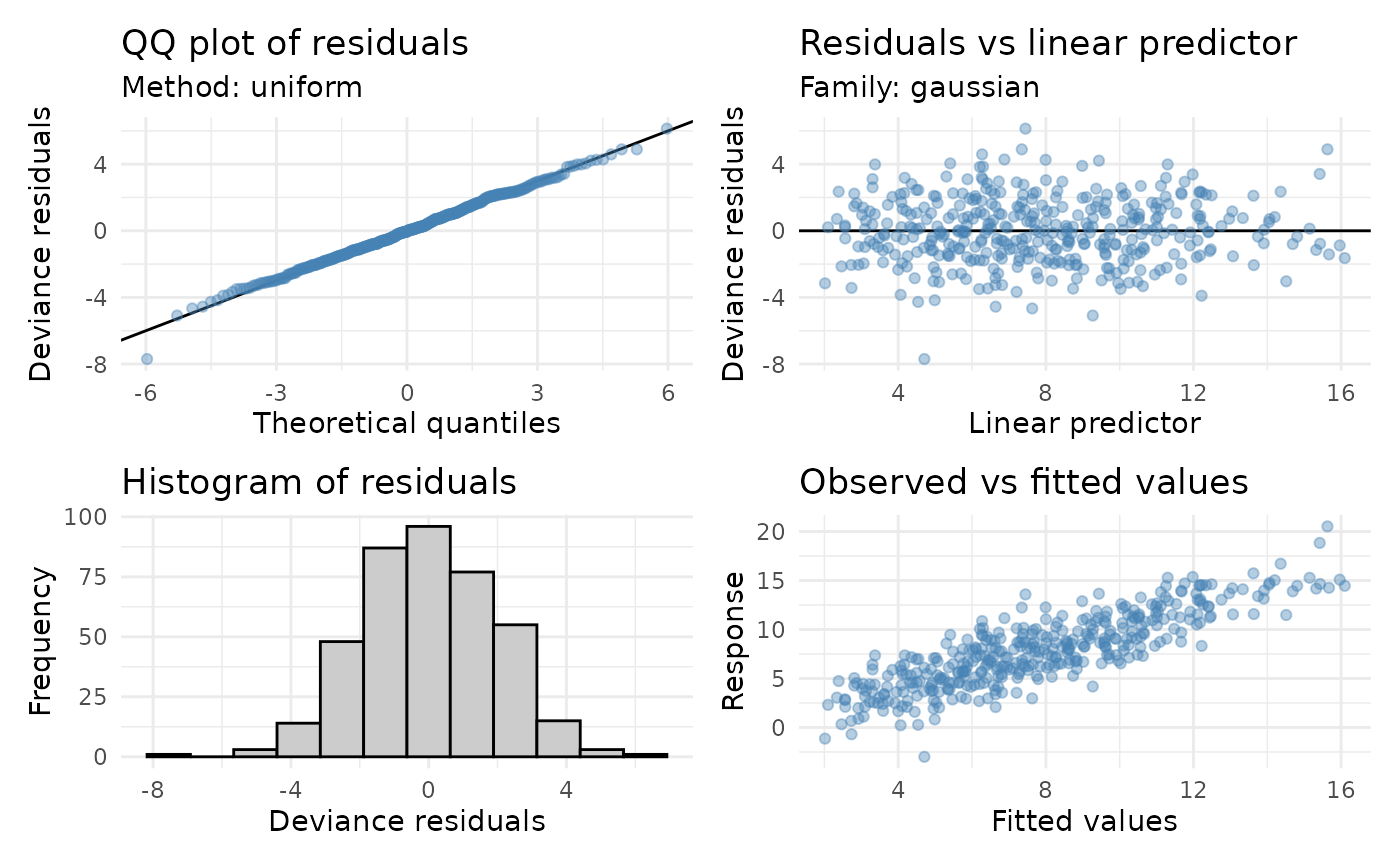Model diagnostic plots

## Usage

appraise(model, ...)

# S3 method for gam
appraise(
model,
method = c("uniform", "simulate", "normal", "direct"),
use_worm = FALSE,
n_uniform = 10,
n_simulate = 50,
type = c("deviance", "pearson", "response"),
n_bins = c("sturges", "scott", "fd"),
ncol = NULL,
nrow = NULL,
guides = "keep",
level = 0.9,
ci_col = "black",
ci_alpha = 0.2,
point_col = "black",
point_alpha = 1,
line_col = "red",
...
)

# S3 method for lm
appraise(model, ...)

## Arguments

model

a fitted model. Currently only class "gam".

...

arguments passed to patchwork::wrap_plots().

method

character; method used to generate theoretical quantiles. Note that method = "direct" is deprecated in favour of method = "uniform".

use_worm

logical; should a worm plot be drawn in place of the QQ plot?

n_uniform

numeric; number of times to randomize uniform quantiles in the direct computation method (method = "direct") for QQ plots.

n_simulate

numeric; number of data sets to simulate from the estimated model when using the simulation method (method = "simulate") for QQ plots.

type

character; type of residuals to use. Only "deviance", "response", and "pearson" residuals are allowed.

n_bins

character or numeric; either the number of bins or a string indicating how to calculate the number of bins.

ncol, nrow

numeric; the numbers of rows and columns over which to spread the plots.

guides

character; one of "keep" (the default), "collect", or "auto". Passed to patchwork::plot_layout()

level

numeric; the coverage level for QQ plot reference intervals. Must be strictly 0 < level < 1. Only used with method = "simulate".

ci_alpha, ci_col

numeric; the level of alpha transparency for the QQ plot reference interval when method = "simulate", or points drawn in plots.

point_col, point_alpha

colour and transparency used to draw points in the plots. See graphics::par() section Color Specification. This is passed to the individual plotting functions, and therefore affects the points of all plots.

line_col

colour specification for the 1:1 line in the QQ plot and the reference line in the residuals vs linear predictor plot.

## Note

The wording used in mgcv::qq.gam() uses direct in reference to the simulated residuals method (method = "simulated"). To avoid confusion, method = "direct" is deprecated in favour of method = "uniform".

The plots are produced by functions qq_plot(), residuals_linpred_plot(), residuals_hist_plot(), and observed_fitted_plot().

## Examples

load_mgcv()
## simulate some data...
dat <- data_sim("eg1", n = 400, dist = "normal", scale = 2, seed = 2)
mod <- gam(y ~ s(x0) + s(x1) + s(x2) + s(x3), data = dat)
## run some basic model checks
appraise(mod, point_col = "steelblue", point_alpha = 0.4)## To change the theme for all panels use the & operator, for example to
## change the ggplot theme for all panels
library("ggplot2")
appraise(mod, point_col = "steelblue", point_alpha = 0.4,
line_col = "black") & theme_minimal()